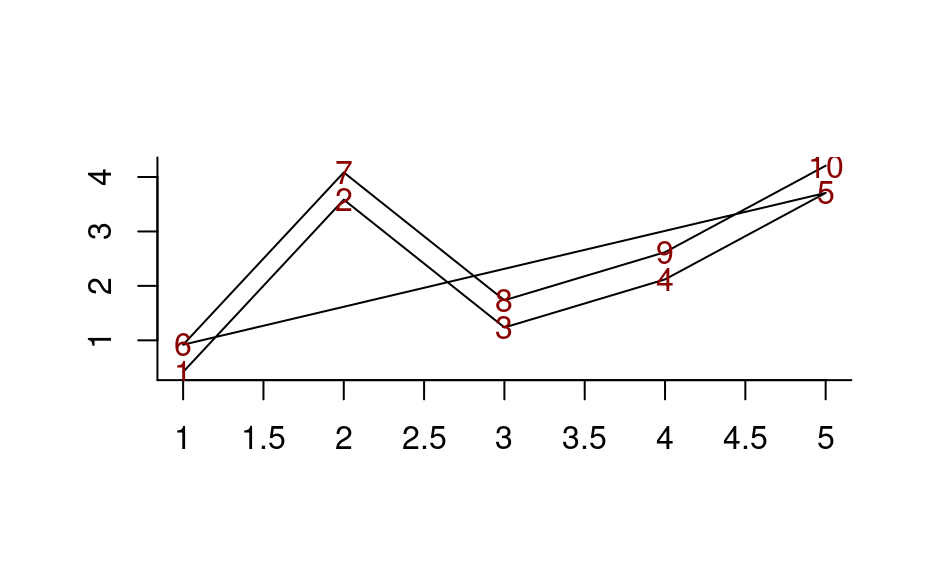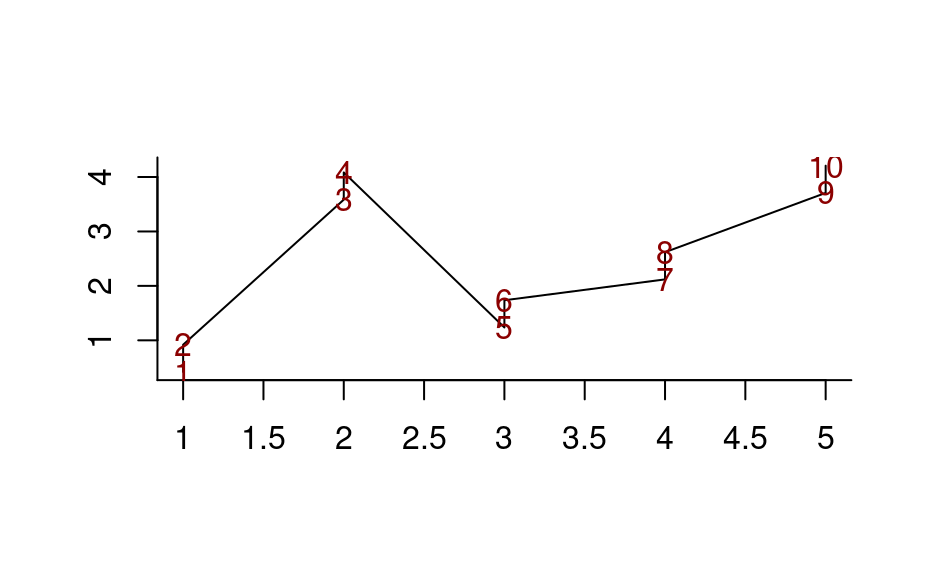Rearranges the hyperSpec object so that the wavelength vector is in increasing (or decreasing) order.

The wavelength vector is sorted and the columns of the spectra matrix are rearranged accordingly.

wl_sort(x, na.last = TRUE, decreasing = FALSE)

Arguments

x

The hyperSpec object.

na.last, decreasing

Handed to base::order().

Value

A hyperSpec object.

C. Beleites

Examples

## Example 1: different drawing order in plot_spc
spc <- new("hyperSpec", spc = matrix(rnorm(5) + 1:5, ncol = 5))
spc <- cbind(spc, spc + .5)

plot_spc(spc)
text(wl(spc), spc[[]], as.character(1:10), col = "darkred")spc_sorted <- wl_sort(spc)
plot_spc(spc_sorted)
text(wl(spc_sorted), spc_sorted[[]], as.character(1:10), col = "darkred")## Example 2
spc <- new("hyperSpec", spc = matrix(rnorm(5) * 2 + 1:5, ncol = 5))
spc <- cbind(spc, spc)

plot(seq_len(nwl(spc)), spc[[]], type = "b")
spc[[]]
#>             1          2        3       4        5        1          2        3
#> [1,] 2.819341 -0.2155114 2.231752 4.16547 4.032235 2.819341 -0.2155114 2.231752
#>            4        5
#> [1,] 4.16547 4.032235

spc_sorted <- wl_sort(spc)
lines(seq_len(nwl(spc_sorted)), spc_sorted[[]], type = "l", col = "red")spc_sorted[[]]
#>             1        1          2          2        3        3       4       4
#> [1,] 2.819341 2.819341 -0.2155114 -0.2155114 2.231752 2.231752 4.16547 4.16547
#>             5        5
#> [1,] 4.032235 4.032235